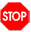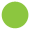04-syntax-semantics

# Module 04: Syntax and semantics

• Write your team names here:

• You may again choose whoever you want to start as the driver. Write your choice here:

Remember:

• Be sure that your module loads into GHCi with no errors before turning it in.
• Write in complete sentences, with capital letters and punctuation. Presentation matters!
``````{-# LANGUAGE GADTSyntax #-}

import Data.Char   -- so you can use the 'isDigit' function later``````

## Some example grammars

Example 1

``````<mirror> ::= '.'
| 'L' <mirror> 'R'``````

Example strings that match `<mirror>`:

``````"."
"L.R"
"LL.RR"``````

Example strings that do not match `<mirror>`:

``````"Q"
"LR"
"LL.RRR"``````

Example 2

``````<tree> ::= '#'
| '(' <tree> ')'
| '(' <tree> <tree> ')'``````

Example strings that match `<tree>`:

``````"(##)"
"((#)(#(##)))"``````

Example strings that do not match `<tree>`:

``````"((#)"
"(###)"``````

Example 3

``````<digit>   ::= '0' | '1' | '2' | '3' | '4' | '5' | '6' | '7' | '8' | '9'
<natural> ::= <digit> | <digit> <natural>
<integer> ::= <natural> | '-' <natural>``````

Example strings that match `<integer>`:

``````"0023"
"-25"``````

Example strings that do not match `<integer>`:

``````"x27"
"--25"
"4-2"``````
• For each of `<mirror>`, `<tree>`, and `<integer>`, give three more examples of strings that match, and three more examples that do not match.

• What does `|` mean? (Note for this question and the next: this is not Haskell syntax! Just say what you think these notations mean based on the examples above.)

• What is the difference between something in single quotes (like ’*’) and something in angle brackets (like `<tree>`)?

The things in single quotes are usually called terminals, and the things in brackets are called nonterminals. These sorts of definitions are known as (context-free) grammars, written in Backus-Naur Form or Backus Normal Form (BNF), named for John Backus and Peter Naur.

• In what context was BNF first developed?

• What else are John Backus and Peter Naur known for?

Now, back to your regularly scheduled grammars…

• Does `<natural>` match the empty string ""? Why or why not?

• An alternative, equivalent way to define `<natural>` is as follows:

``  <natural> ::= <digit>+``

Given that this is equivalent to the original definition, what do you think `+` means?

Technically this sort of `+` notation was not included in the original form of BNF, but it is a common extension.

• `<natural> ::= <digit>*` would match all the same strings as `<digit>+`, but also matches the empty string. What do you think `*` means in this context?

• Describe how to modify the definition of `<natural>` so it does not allow unnecessary leading zeroes. That is, `"203"` should still match but `"0023"` should not; however, `"0"` should still be a valid `<natural>`. If you wish, you can also introduce more definitions or modify definitions besides `<natural>`.

• Write down a grammar (as concisely as possible) that matches all these strings:

``````  "(XX)Y"
"(XXXX)YZ"
"(XX)ZZZZZ"
"(XXXXXX)YZZ"``````

…but does not match any of these strings:

``````  "()Y"
"(XXX)YZ"
"(X)ZZZ"
"(XX)YY"``````## Mirror, mirror

• ROTATE ROLES and write the name of the new driver here:
``````{-
<mirror> ::= '.'
| 'L' <mirror> 'R'
-}

data Mirror where
Middle :: Mirror
Layer  :: Mirror -> Mirror
deriving Show

prettyMirror :: Mirror -> String
prettyMirror Middle    = "."
prettyMirror (Layer m) = "L" ++ prettyMirror m ++ "R"

parseMirror :: String -> (Mirror, String)
parseMirror ('.' : rest) = (Middle, rest)
parseMirror ('L' : rest) =
case parseMirror rest of
(m, 'R' : rest') -> (Layer m, rest')``````
• Write down three different example values of type `Mirror`.

• Try calling `prettyMirror` on your example `Mirror` values above, and record the results.

• For this language, how are the concrete syntax (represented by the grammar `<mirror>`) and abstract syntax (represented by the data type `Mirror`) different?

• Try calling `parseMirror` on five different example inputs. An example input can be any `String`. Try to pick a variety of examples that show the range of behavior of `parseMirror`. Record the results here.

• Describe the behavior of `parseMirror`. Your answer should refer to the grammar `<mirror>`.

• Why does `parseMirror` return a `(Mirror, String)` pair instead of just a `Mirror`? (Hint: if you are not sure, try writing a function `parseMirror2 :: String -> Mirror` which behaves the same as `parseMirror` but does not return the extra `String`.)

• Modify `parseMirror` so that it has type `String -> Maybe (Mirror, String)` and never crashes. Instead of crashing on inputs that do not match `<mirror>`, it should return `Nothing`. Call your modified function `parseMirrorSafe` and write it below.## BIN (aka Your First Language)

• ROTATE ROLES and write the name of the new driver here:

Consider the following BNF grammar:

``````<bin> ::= '#'
| '(' <bin> <bin> ')'``````
• Write an algebraic data type called `Bin` which corresponds to `<bin>`. That is, `Bin` should encode the abstract syntax trees corresponding to the concrete syntax `<bin>`.

• Write a function `prettyBin` which turns an abstract syntax tree into concrete syntax.

• Write a function `parseBin :: String -> Maybe (Bin, String)` which turns concrete syntax into abstract syntax.

One way we could give a semantics (meaning) to abstract `Bin` trees is as follows: a leaf has value 1; the value of a branch with left and right subtrees is the value of the left subtree plus twice the value of the right subtree.

• Write a function `interpBin :: Bin -> Integer` which implements this semantics.

We can think of this as a little programming language for computing integers; let’s call it BIN. (Of course, BIN is not a very useful language, but don’t let it hear you say that because you might hurt its feelings.) For example, `"((##)(##))"` is a program to compute the value 9. `parseBin` is a parser for the language, and `interpBin` is an interpreter.

• Put the pieces together to create a function `evalBin :: String -> Maybe Integer`. For example,

``````  evalBin "#"          --> Just 1
evalBin "((##)(##))" --> Just 9
evalBin "(##"        --> Nothing``````